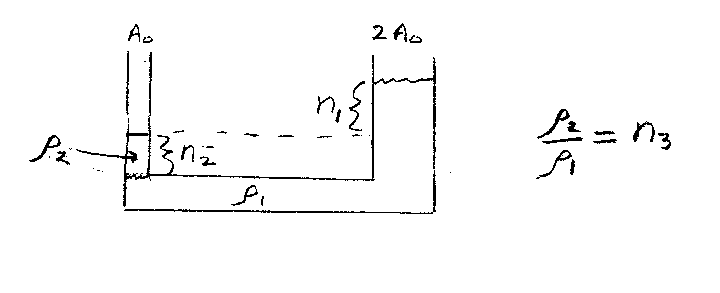Problem A1: Consider the U-shaped tube shown below. The tube contains two different fluids, one in the left column with a density ρ2 and one in the right column with a density ρ1. The fluid in the left column is a height n2 above the bottom piece, and the fluid in the right column is n1 above the left fluid (ρ2). The area of the left column is A0, and the area of the right column is 2A0. If the ratio of densities ρ2 / ρ1 = n3, what is n3?n1 = n2 = Input n3:

If you are currently in my class, you can record your grade by entering your name and student ID number (without the leading zeros) below and clicking on "record grade".
 First Name = Last Name = ID = Problem: